# Mersenne number for prime or Poulet implies prime or Poulet

## Statement

Supposeis a natural number such that:.

Consider theMersenne number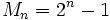. Then, we have: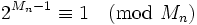.

In other words, the Mersenne number for a number that is either a prime number or a Poulet number (i.e., an odd composite number that is pseudoprime to base), is also either a prime number or a Poulet number.

## Proof

Given: A natural numbersuch that.

To prove:.

Proof: By assumption,divides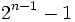, so there exists an integersuch that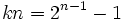. Thus,. Thus, we have: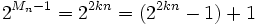.

Sincedivides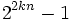, we get that:.